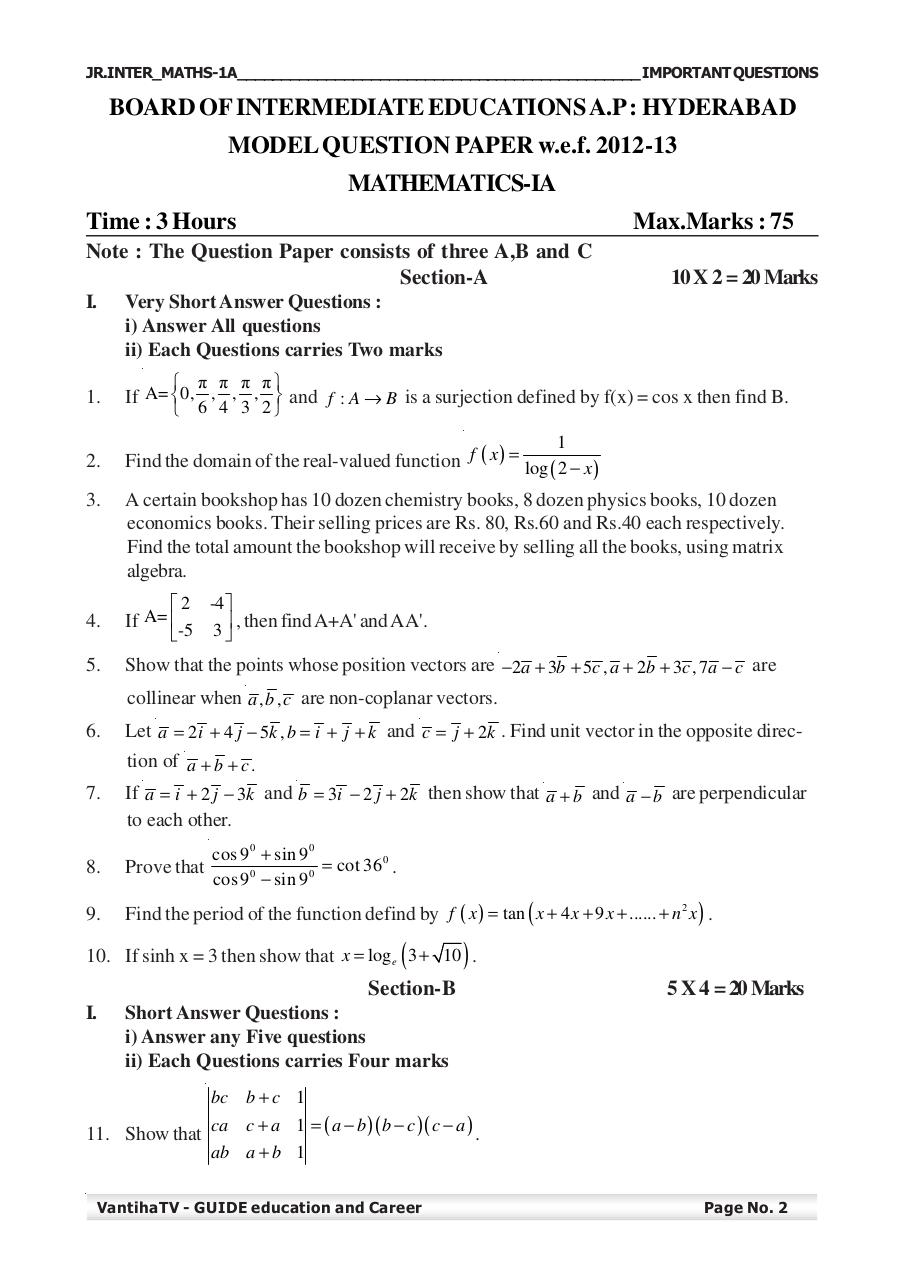# Maths 1A Chapter wise important Questions.pdfPage 1 23427

#### Text preview

JR.INTER_MATHS-1A_____________________________________________ IMPORTANT QUESTIONS

BOARD OF INTERMEDIATE EDUCATIONS A.P : HYDERABAD
MODEL QUESTION PAPER w.e.f. 2012-13
MATHEMATICS-IA
Time : 3 Hours

Max.Marks : 75

Note : The Question Paper consists of three A,B and C
Section-A

10 X 2 = 20 Marks

I.

Very Short Answer Questions :
i) Answer All questions
ii) Each Questions carries Two marks

1.

If A= 0, , , ,  and f : A → B is a surjection defined by f(x) = cos x then find B.
6 4 3 2

2.

Find the domain of the real-valued function f ( x ) = log ( 2 − x )

3.

A certain bookshop has 10 dozen chemistry books, 8 dozen physics books, 10 dozen
economics books. Their selling prices are Rs. 80, Rs.60 and Rs.40 each respectively.
Find the total amount the bookshop will receive by selling all the books, using matrix
algebra.

4.

If A=  -5 3  , then find A+A' and A A'.

5.

Show that the points whose position vectors are −2a + 3b + 5c , a + 2b + 3c , 7a − c are

 π π π π

1

2

-4 

collinear when a , b , c are non-coplanar vectors.
6.

Let a = 2 i + 4 j − 5k , b = i + j + k and c = j + 2k . Find unit vector in the opposite direction of a + b + c .

7.

If a = i + 2 j − 3k and b = 3i − 2 j + 2k then show that a + b and a − b are perpendicular
to each other.

8.

Prove that

9.

Find the period of the function defind by f ( x ) = tan ( x + 4 x + 9 x + ...... + n 2 x ) .

cos 9 0 + sin 9 0
= cot 36 0 .
0
0
cos 9 − sin 9

(

)

10. If sinh x = 3 then show that x = log e 3 + 10 .

Section-B
I.

5 X 4 = 20 Marks

Short Answer Questions :
i) Answer any Five questions
ii) Each Questions carries Four marks
bc b + c 1

11. Show that ca c + a 1 = ( a − b )( b − c )( c − a ) .
ab a + b 1

VantihaTV - GUIDE education and Career

Page No. 2